Volume 5, Issue 6, December 2016, Pages: 57-65

Finite Element Study on the Vibration of Functionally Graded Beam with Different Temperature Conditions

Kazem Reza Kashyzadeh1, *, Alireza Amiri Asfarjani2

1Young Researchers and Elite Club, Semnan Branch, Islamic Azad University, Semnan, Iran

2Department of Mechanical Engineering, Qom University, Qom, Iran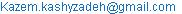(K. R. Kashyzadeh)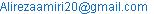(A. A. Asfarjani)

*Corresponding author

Kazem Reza Kashyzadeh, Alireza Amiri Asfarjani. Finite Element Study on the Vibration of Functionally Graded Beam with Different Temperature Conditions. Advances in Materials. Vol. 5, No. 6, 2016, pp. 57-65. doi: 10.11648/j.am.20160506.11

Received: September 10, 2016; Accepted: October 27, 2016; Published: December 23, 2016

Abstract: Functional graded materials have been wide range of applications in the different industries such as automotive, machine, aerospace and etc. So, the vibration analysis under different conditions is very important. FGMs are made of a ceramic and a metal to protect against large temperature gradients. The main objective of this research is to study of the effect of temperature field on the natural frequencies of functional graded (FG) beams with different conditions. The finite element model has been simulated in ANSYS. It is assumed that the beam is made of ceramic and metal, and the effective material properties such as Young’s modulus, Poisson’s ratio and etc. are temperature-dependent and vary continuously through the thickness direction according to a power-law distribution. Natural frequencies have been obtained with different conditions in the environmental temperature. Thus, modal analysis has been performed for a FGM beam with C-C and C-F supports. The obtained results have been compared with other published papers. It has a good agreement. Then, the effect of temperature field and slenderness ratio have been studied on the frequency values of FG thick beams. It is found that the natural frequency of the system is reduced by temperature increasing under all support conditions. And it is reduced by decreasing zirconia material at every constant temperature.

Keywords: Natural Frequency, FGM Beam, Temperature Field, Slenderness Ratio, Finite Element Method

Contents

1. Introduction

During the last few years, functional graded materials (FGMs) have been used more and more in the various industries such as biomechanics, automotive, aerospace and naval and etc. FG materials are one of the most practical materials that used in industry with high temperature environment.

A common type of this materials is composed of metal and ceramic constituents which is obtained by mixing the powder [1-3]. On the other hand, metal and ceramic phases continuously change from one level to another. It means that one level of material is pure ceramic and another level is pure metal. So, between two levels is the composition of the two phases such as shown in Figure 1.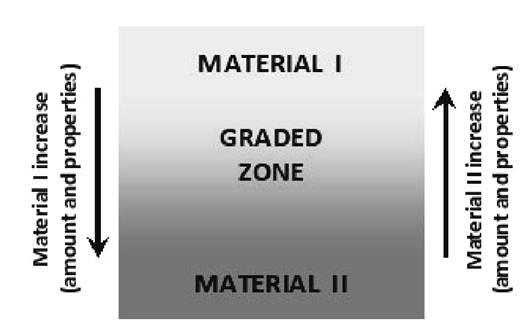Fig. 1. Idea of functionally graded materials .

The mechanical properties change in the thickness direction according to the type of material combinations.

The first finding of these materials was related to their application in the thermal environment. In the 1980s, FGMs were considered in Japan for thermal barrier coatings protecting the space plane from high surface temperature and withstanding a high thermal gradient through the thickness [1, 2, and 5]. But, nowadays the main applications of these materials are:

·     Construction of the reactor wall

·     Applications of medical engineering such as dental implants or synthetic bones

·     The use of ceramic engines to resist to the corrosion in the high temperature

·     Solid oxide fuel cells

Sandwich panels with graded facing-core interfaces

The study of the FGMs’ behavior under different loadings and temperature conditions due to their widespread use in various industries is very important. Several researchers have studied the behavior of these materials and this materials have been analyzed in different phenomena.

Low and high velocity impact response of FGM beam with general boundary has been studied by experimental, finite element and theoretical method [6-11]. Other researchers have developed the results. And the structures have been optimized to absorb more impact energy and to reduce impact damage.

Shen has presented static post-buckling analysis for FGM cylindrical shells with piezoelectric actuators subjected to the different loadings and various conditions [12-14]. Shariyat has studied the dynamic buckling of suddenly loaded in the FGM cylindrical shells with temperature-dependent material properties under thermo-electro-mechanical loads .

By increasing use of beams as structural components in various fields such as civil and aerospace engineering, the study of vibration behavior of FGM beams has necessitated and a large number of studies can be found in literature about beam vibrations.

Investigation on the rotating beams has been presented by number of researchers [16-19]. Kapuria et al. have studied vibration response of layered FG beams . Li et al. have suggested new approach for modal analyzing of Euler and Timoshenko FG beams . Simsek has studied free vibration response of FG beam for different higher order beam theories . Pradhan et al. have evaluated natural frequencies of Euler and Timoshenko FG beams under various boundary conditions . Natural frequencies of FGM beams have been calculated by using numerical methods [24-25]. The free vibration and bending of FGM beams resting have been studied on elastic foundation . Forced vibration of FGM Timoshenko beam with piezoelectric layers carrying moving load have been investigated to develop vibration behaviour of FGM beams 

In the Present work, the effect of temperature field on the natural frequencies of FG beams under different boundary conditions has been studied by using finite element method. The material properties change continuously through the thickness direction according to a power-law distribution. Afterward, the effects of slenderness ratio (L/H) on the results is discussed.

2. Material Properties

Mechanical properties of FGM materials change continuously from one level to another. And it also depends on the combination of FGM. Functional graded materials usually are formed from the combination of ceramics and metals. Ceramics with special high temperature properties are combined with metals because of increasing mechanical characteristics. According to the rule of mixture, the effective material properties, P, can be expressed as :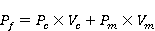(1)

Where c and m indexes are related to ceramic and metal respectively. And Vm and Vc are the volume fractions of the components. Thus, we have :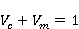(2)

Mechanical properties of FGMs such as elasticity modulus, Poisson's ratio and density can be calculated as :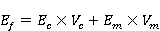(3)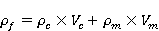(4)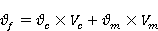(5)

These are the results of mixture law for composite materials. So, FGMs can be regarded as a type of advanced composite materials. Material properties formulas can be rewritten as :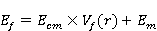(6)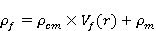(7)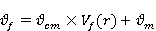(8)

Where :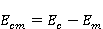(9)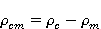(10)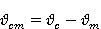(11)

And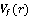is the volume fraction distribution of power, which is defined as follows :(12)

The positive value of parameter N (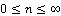) represents profile of material changes in the thickness direction. The FG beam becomes a fully metal beam when N is set to 2 in this research and becomes a fully ceramic beam when N is zero. On the other hand, ceramic properties reduce and metal properties increase in the beam by increasing the number of N. From the above relationship, the material properties are expressed as: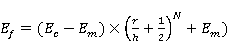(13)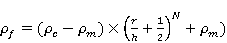(14)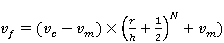(15)

Functional graded materials also are used in high temperature environments. The material properties will have significant changes in high temperature. So, it is essential to account this temperature-dependence for studying behavior of the structural. Farid and et al. have extracted a non-linear function of temperature as :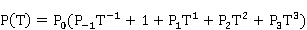(16)

Where T is working temperature in kelvin and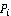is temperature dependent coefficient that is unique for any materials.

In the present work, the FGM beam has been composed of zirconia (ZrO2) and Stainless steel (SUS304) in which mechanical properties are shown in Table.1 .

Table 1. Mechanical properties of functional graded beam components .

 Material Density (Kg/m3) Elastic Modulus (GPa) Poisson’s Ratio Zirconia 5700 244.27 0.288 Stainless steel 8166 201.04 0.3262

The temperature coefficients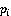are given according to Shariyat , and the effect of temperature on the material properties have been presented in Figure 2.

3. Finite Element Simulation

The rectangular beam was modelled with the following parameters: Length=20m, width= 1m and thickness=1m. The thickness section is composed from 10 layers with equal dimensions. Zirconia and stainless steel material is assigned to all layers when the number of N is set to zero and 2 respectively. So, when N is equal one, each material is assigned to half of layers.

The edge length and width are divided to 50 and 5 numbers respectively. And thickness of each layers include 2 number of divisions to simulate finite element model. The final FEM of functional graded beam has 2500 elements as shown in Figure 3.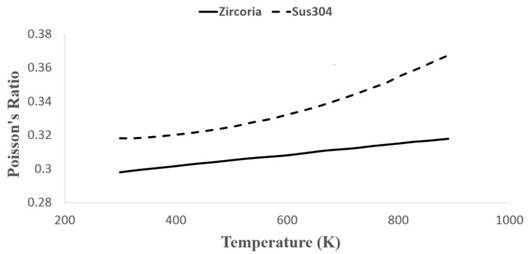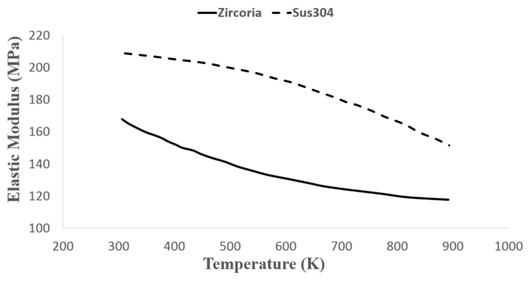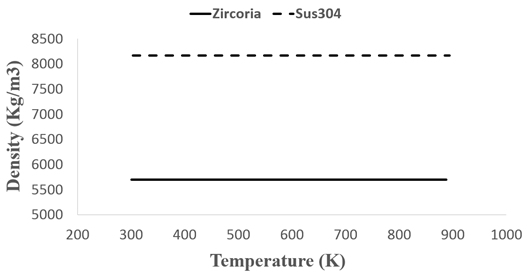Fig. 2. The effect of temperature on the material properties .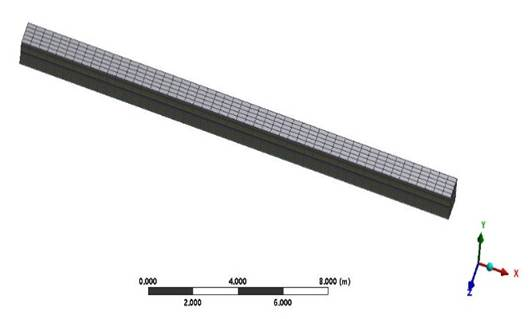Fig. 3. Finite element model of FGM beam.

3.1. Effects of Material Distribution

Modal analysis has been performed for double clamp support FGM beam to evaluated natural frequencies. This analysis is considered to room temperature (T=300K). The first six natural frequencies of FGM beam with different number of N is reported in Table. 2 and shown as Figure 4.

Table 2. The first six natural frequencies of FGM with different number of N at room temperature and L/H=20.

 Frequency Set (Hz) Number of N 0 0.2 1 2 1 2.2785 2.1733 2.0981 2.112 2 2.281 2.066 2.127 2.012 3 2.485 2.378 2.318 2.164 4 3.661 3.492 3.373 3.227 5 3.668 3.511 3.42 3.234 6 4.336 4.153 4.044 3.826

It is clear from figure 3 that the highest frequencies are considered to the pure ceramic beam. And so on, the lowest values are evaluated for pure stainless steel beam. Also natural frequencies decrease by increasing number of N parameter. This difference is not obvious at the first set of frequency but it increase gradually. This results have been extracted for FGM beam at room temperature. Besides, natural frequencies also increase in each group which can represent FEM is correct.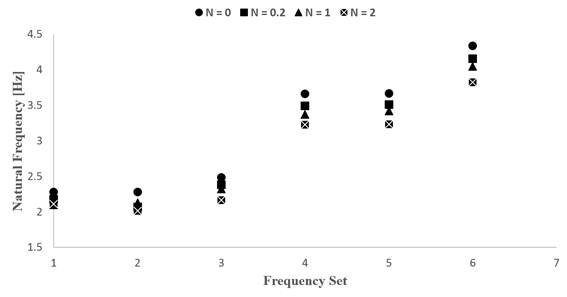Fig. 4. The first six natural frequencies of FGM with different number of N at room temperature and L/H=20.

3.2. Effects of Temperature

In this section, the effects of high temperature has been studied on the vibration behaviour of FGM beam with C-C supports. Modal analysis has been performed for different values of N. the results for different temperatures of 450, 600, 750 and 900K have been reported in Tables 3, 4, 5 and 6 respectively.

Table 3. The first six natural frequencies of FGM with different number of N at T=450K and L/H=20.

 Frequency Set (Hz) Number of N 0 0.2 1 2 1 2.104 2.066 2.036 2.074 2 2.107 2.089 2.079 2.077 3 2.291 2.259 2.254 2.242 4 3.381 3.319 3.276 3.332 5 3.388 3.358 3.342 3.338 6 4.0055 3.9722 3.953 3.949

Table 4. The first six natural frequencies of FGM with different number of N at T=600K and L/H=20.

 Frequency Set (Hz) Number of N 0 0.2 1 2 1 1.9929 1.9773 1.9651 2.0099 2 1.9956 2.0056 2.0113 1.994 3 2.1657 2.1576 2.1706 2.1235 4 3.2018 3.1769 3.1609 3.2149 5 3.2082 3.2235 3.2323 3.1742 6 3.7932 3.8129 3.8241 3.6566

Table 5. The first six natural frequencies of FGM with different number of N at T=750K and L/H=20.

 Frequency Set (Hz) Number of N 0 0.2 1 2 1 1.9347 1.9036 1.8794 1.916 2 1.9374 1.9262 1.92 1.935 3 2.0987 2.068 2.0622 2.0703 4 3.1081 3.058 3.022 3.1028 5 3.1143 3.0954 3.0847 3.1089 6 3.6827 3.4929 3.4209 3.4051

Table 6. The first six natural frequencies of FGM with different number of N at T=900K and L/H=20.

 Frequency Set (Hz) Number of N 0 0.2 1 2 1 1.8991 1.825 1.7705 1.7881 2 1.9017 1.8364 1.7988 1.7905 3 2.0563 1.9708 1.923 1.8977 4 3.0506 2.9308 2.8448 2.8699 5 3.0567 2.9507 2.8893 2.8755 6 3.615 3.4929 3.4209 3.4051

Moreover, Comparison curves of first, second and third natural frequencies of FGM beam with C-C supports for different temperatures have been plotted in Figures 5, 6 and 7 respectively.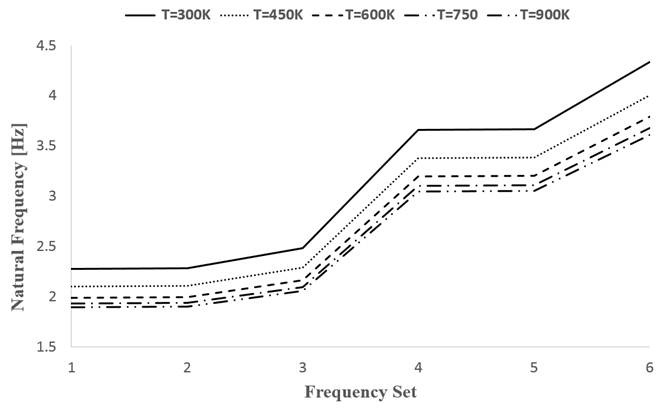Fig. 5. The first natural frequency of FGM with N is set to zero in the various temperature and L/H=20.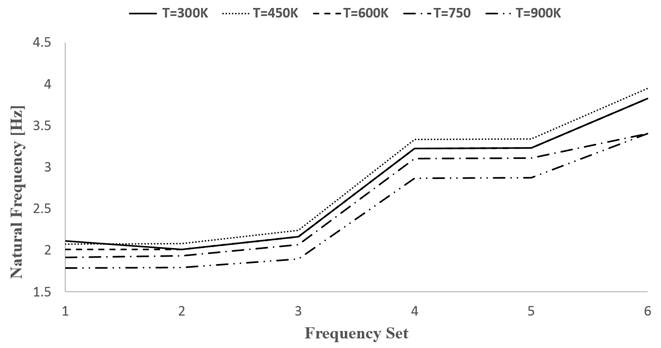Fig. 6. The first natural frequency of FGM with N is set to 2 in the various temperature and L/H=20.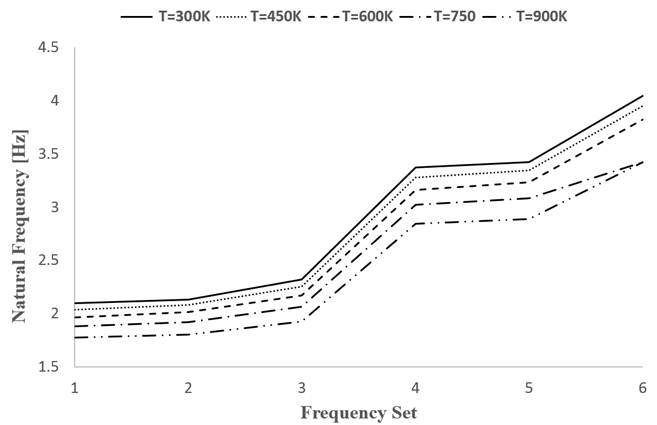Fig. 7. The first natural frequency of FGM with N=1 in the various temperature and L/H=20.

It is obvious that the frequencies are reduced by temperature increasing based on all the obtained results. This is true for all values of the parameter N. It can be derived from the tables that the frequencies will be decreased by increasing number of N. But the results seem to be not normal when N=2 and the highest frequency is related to the temperature of 450 Kelvin. Then, downward trend has continued. This abnormal behavior not seen in the other parts of study.

3.3. Effects of Slenderness Ratio (L/H)

Three different models have been used for modal analysis to investigate the effect of slenderness ratio of L/H = 5, 10 and 20. The environment temperature is assumed of 300K for all analyses. Finally, the FEM results of frequencies are reported in the following tables and comparison curve is plotted for N=1.

Table 7. The first six natural frequencies of FGM with different number of N at room temperature and L/H=5.

 Frequency Set (Hz) Number of N 0 1 2 1 5.8524 5.583 5.4231 2 5.8769 5.6226 5.4457 3 10.005 9.5818 9.2089 4 13.45 12.825 12.441 5 13.538 12.934 12.522 6 17.483 16.742 16.227

Table 8. The first six natural frequencies of FGM with different number of N at room temperature and L/H=10.

 Frequency Set (Hz) Number of N 0 1 2 1 1.697 1.6193 1.5746 2 1.6994 1.6279 1.5767 3 4.3785 4.1772 4.059 4 4.3912 4.2039 4.0707 5 4.9821 4.7684 4.5855 6 7.9818 7.6136 7.3934

Table 9. The first six natural frequencies of FGM with different number of N at room temperature and L/H=20.

 Frequency Set (Hz) Number of N 0 1 2 1 2.2785 2.0981 2.1122 2 2.2817 2.1275 2.0126 3 2.485 2.3185 2.1641 4 3.6613 3.3737 3.2278 5 3.6686 3.42 3.2342 6 4.3364 4.0442 3.8265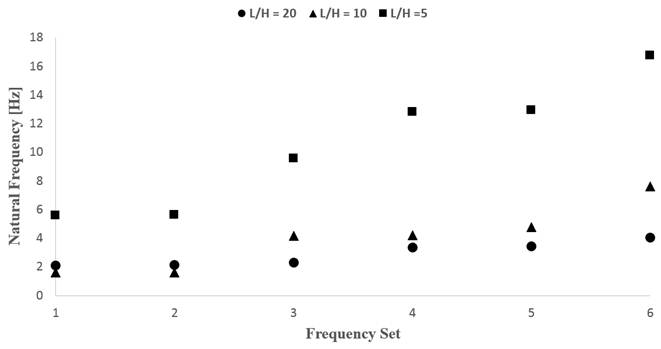Fig. 8. Comparison curve of frequencies with N=1 in the room temperature and different slenderness ratio of L/H.

The values of natural frequencies reduce by increasing aspect ratio of L/H. So, the highest and lowest frequencies are related to the slenderness ratio of 5 and 20 respectively. However, there is no direct relationship between them. On the other hand, if use of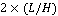, cannot be concluded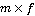.

3.4. Effects of Different Conditions

Vibration analysis has been performed for FGM beam with different boundary conditions such as C-C and C-F supports. The analysis was done at room temperature (300K) and with aspect ratio of 10 for L/H.

Table 10. The first six natural frequencies of FGM with different boundary conditions at room temperature and L/H=10.

 Frequency Set (Hz) Number of N N=0 N=1 N=2 C-C C-F C-C C-F C-C C-F 1 1.697 0.27899 1.6193 0.26614 1.5746 0.25879 2 1.6994 0.27899 1.6279 0.26725 1.5767 0.25879 3 4.3785 1.6785 4.1772 1.6013 4.059 1.5563 4 4.3912 1.6801 4.2039 1.6088 4.0707 1.5577 5 4.9821 2.485 4.7684 2.3782 4.5855 2.2871 6 7.9818 4.3364 7.6136 4.1524 7.3934 4.0208

The natural frequencies are reduced by increasing number of N in the FGM C-F beam. The frequencies of beam with a free edge are also less than beam with supports.

4. Conclusions

In the present paper, finite element method has been exploited to study the vibration behaviour of FGM beam. The material properties were assumed to be temperature-dependent and varied continuously thorough the thickness according to power-law form. Finally the effects of high temperature, slenderness ratio and different boundary conditions have been studied on the natural frequencies of FGM beam. Obtained results show that:

1.   The highest frequencies are considered to the pure ceramic beam. And the lowest values are evaluated for pure stainless steel beam.

2.   The natural frequencies also decrease by increasing number of N parameter.

3.   The values of natural frequencies are reduced by increasing temperature and this is true for all values of the parameter N.

4.   The natural frequencies are reduced by increasing slenderness ratio of L/H. So, the highest and lowest frequencies are related to the slenderness ratio of 5 and 20 respectively. However, there is no direct relationship between them. On the other hand, if use of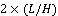, cannot be concluded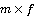.

5.   The natural frequencies are reduced by increasing number of N in the FGM beam with C-F support.

6.   The frequencies of beam with a free edge are also less than beam with simply supports.

References

1. Mahamood R. M, Akinlabi E. T, Shukla M, Pityana S, (2012), "Functionally graded material: An overview", Proceedings of the World Congress on Engineering, London, U. K., Vol. 3.
2. EL-Wazery M. S, EL-Desouky A. R, (2015), "A review on Functionally Graded Ceramic-Metal Materials", Mater. Environ. Sci., Vol. 6, Issue. 5.
3. Hasani sadi M, (2014), "Functionally graded materials and manufacturing methods", Journal of Manufacturing Engineering, Vol. 45, (in Persian).
4. Cho J. R, Tinsley Oden J, (2000), "Functionally graded material: a parametric study on thermal stress characteristics using the Crank-Nicolson-Galerkin scheme", Comput. Methods. Appl. Mech. Engrg. Vol. 188.
5. Wosko M, Paszkiewicz B, Piasecki T, Szyszka A, (2005), "Application and modeling of functionally graded materials for optoelectronic devices", IEEE.
6. Kiani Y, Sadighi M, Jedari Salami S, Eslami M. R, (2013), "Low velocity impact response of thick FGM beams with general boundary conditions in thermal field", Composite Structures, Vol. 104.
7. Zamani J, Etemadi E, Hosseini Safari K, Afaghi Khatibi A, (2014), "Modeling of High Velocity Impact in Sandwich Beams with FGM Core", Series of Advanced Structured Materials Book, Vol. 35.
8. Mohammadi Y, Khalili S. M. R, Malekzadeh Fard K, (2016), "Low velocity impact analysis of sandwich plates with functionally graded face sheets", Mechanics of Advanced Materials and Structures, Vol. 23, Issue. 4.
9. Safri S. N. R, Sultan M. T. H, Yidris N, Mustapha F, (2014), "Low Velocity and High Velocity Impact Test on Composite Materials-A Review", International Journal of Engineering and Science, Vol. 3, Issue. 9.
10. Fu Y, Hu S, Mao Y, (2016), "Nonlinear low velocity impact analysis of functionally graded shallow spherical shells in thermal environments", Journal of Thermoplastic Composite Materials.
11. Asemi K, Jedari Salami S, (2015), "A study on low velocity impact response of FGM rectangular plates with 3D elasticity based graded finite element modeling", Journal of Theoretical and Applied Mechanics, Vol. 53, Issue. 4.
12. Shen H, Leung A, (2003), "Postbuckling of pressure loaded functionally graded cylindrical panels in thermal environments", Journal of Engineering Mechanics, Vol. 129.
13. Shen H, (2004), "Thermal postbuckling behavior of functionally graded cylinder shells with temperature dependent properties", International Journal of Solids and Structures, Vol. 41.
14. Shen H, Noda N, (2005), "Postbuckling of FGM cylindrical shells under combined axial and radial mechanical loads in thermal environments", International Journal of Solids and Structures, Vol. 42.
15. Shariyat M, (2008), "Dynamic buckling of suddenly loaded imperfect hybrid FGM cylindrical shells with temperature dependent material properties under thermo-electro-mechanical loads", International Journal of Mechanical Science, Vol. 50.
16. Hodges, Yang D, Yang Rutkowski M, (1981), "Free-vibration analysis of rotating beams by a variable order finite element method", AIAA Journal, Vol. 19, Issue. 11.
17. Mei C, (2008), "Application of differential transformation technique to free vibration analysis of a centrifugally stiffened beam", Computers & Structures, Vol. 86.
18. Mohanty S. C, Dash R. R, Rout T, (2013), "Free Vibration of a Functionally Graded Rotating Timoshenko Beam Using FEM", Advances in Structural Engineering, Vol. 16.
19. Ebrahimi F, Mokhtari M, (2015), "Semi-analytical vibration characteristics of rotating Timoshenko beams made of functionally graded materials", Latin American Journal of Solids and Structures, Vol. 12.
20. Kapuria S, Bhattacharyya M, Kumar A, (2008), "Bending and free Vibration response of layered functionally graded beams, a theoretical model and its experimental validation", Composite Structures, Vol. 82, Issue. 3.
21. Li X. F, Kang Y. A, Wu J. X, (2013), "Exact frequency equations of free vibration of exponentially functionally graded beams", Applied Acoustics, Vol. 74, Issue. 3.
22. Şimşek M, (2010), "Fundamental frequency analysis of functionally graded beams by using different higher-order beam theories", Nuclear Engineering and Design, Vol. 240.
23. Pradhan K. K, Chakraverty S, (2013), "Free vibration of Euler and Timoshenko functionally graded beams by Ray-leigh–Ritz method", Composites Part B: Engineering, Vol. 51.
24. Arjangpay A, Ansari R, Darvizah M, (2012), "Vibration analysis of a FGM cylindrical shell using MLPG method",Modares Mechanical Engineering, Vol. 13, Issue. 3, (in Persian).
25. Shirong L, Liange F, (2014), "Free vibration of FGM Timoshenko beams with through width delamination", Science China Physics, Mechanics & Astronomy, Vol. 57.
26. Akbas S. D, (2015), "Free vibration and bending of functionally graded beams resting on elastic foundation", Research on Engineering Structures & Materials, Vol. 1.
27. Jafari A. A, Fathabadi M, (2012), "Forced vibration of FGM Timoshenko beam with piezoelectric layers carrying moving load", Journal of Aerospace and Mechanic Engineering, Vol. 9, Issue. 2.
28. Farid M, Zahedinejad P, Malekzadeh P, (2010), "Three-dimensional temperature dependent free vibration analysis of functionally graded material curved panels resting on two-parameter elastic foundation using a hybrid semi-analytic, differential quadrature Method", Materials & Design.
29. Alshorbagy A. E, (2013), "Temperature effects on the vibration characteristics of a functionally graded thick beam", Ain Shams Engineering Journal, Vol.4.

 Contents 1. 2. 3. 3.1. 3.2. 3.3. 3.4. 4.
Article ToolsAbstractPDF(758K)